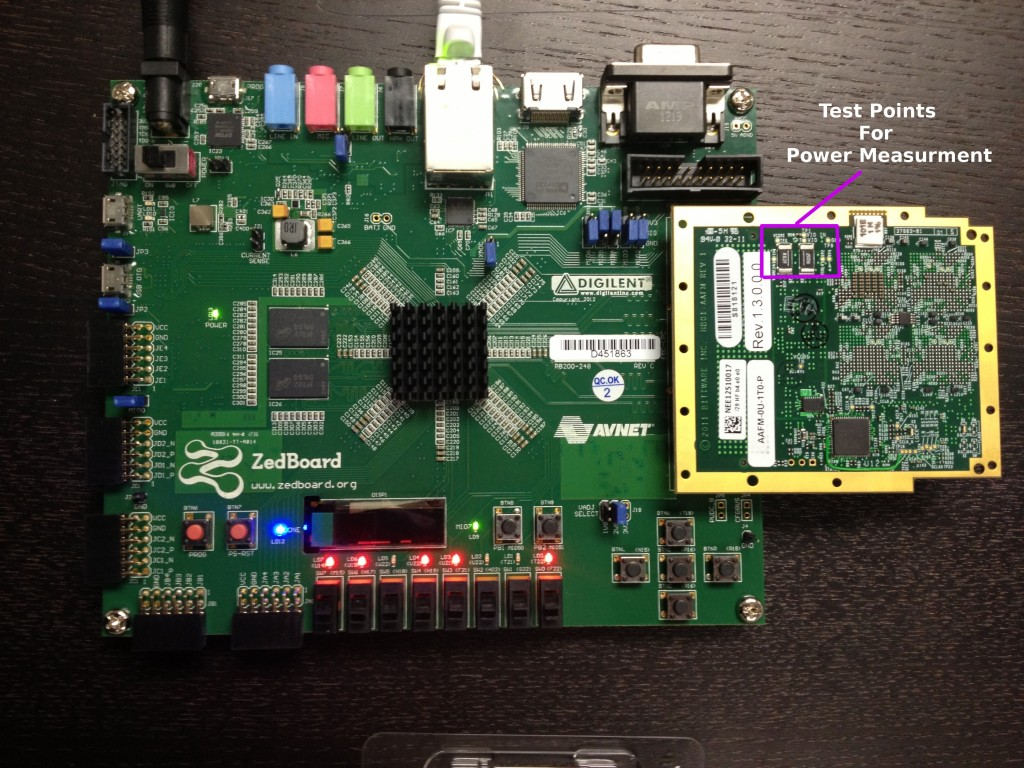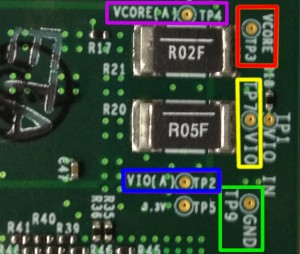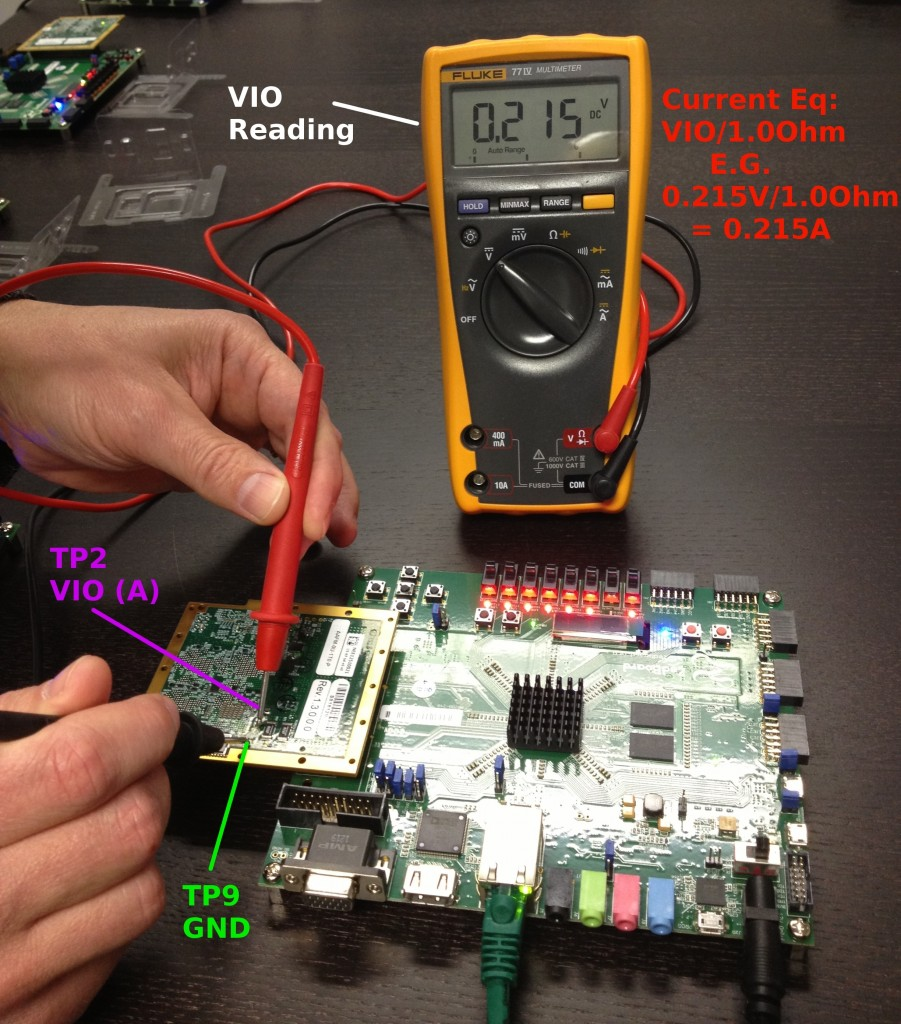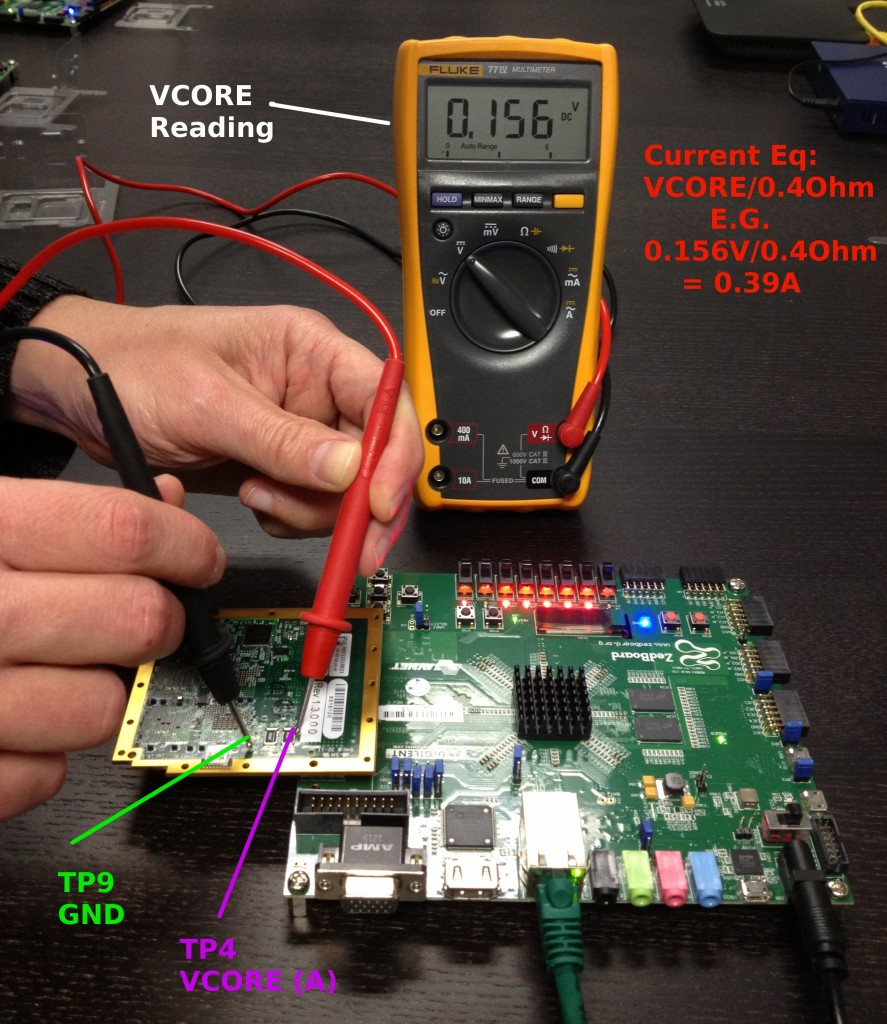# Measuring Power Consumption on the Parallella Prototype Board

One of the central focuses of the Epiphany architecture and the Parallella platform is energy efficient computing. We understand that many developers are interested in measuring the power consumption of their applications running on the Epiphany chips. For this purpose the Parallella board contains “power measurement” test points the usage of which is described in this application note. No soldering or board modification is needed, but you will need a digital multimeter.

So, how do we measure Epiphany chip power?

The measurement of the power consumption is achieved by measuring the voltage and the current driven to the chip. Epiphany chips have two separate power domains – IO power domain and core power domain. After measuring the voltage and current of each domain according to the explanation provided below, one can calculate the power consumed by chip’s IO and core based on the following equations:

Pio = Vio×Iio

Pcore=Vcore×Icore

The following picture shows Parallella prototype platform with the “power measurement” on the Epiphany based FMC daughter card area highlighted in purple.Next is a closer look at the “power measurement” area:What do we see here ?

• TP9 -GND (highlighted in green). Ground plane test point.
• TP2-VIO(A) (highlighted in blue). Test point used to measure voltage across the IO current sense resistor (1.0 Ohm). Once the voltage across this resistor is known the current is calculated by dividing the measured voltage by 1.0 Ohm.
• TP4-VCORE(A) (highlighted in purple). Test point used to measure voltage across the current sense  resistor (0.4 Ohm). We will calculate the core current by dividing the voltage measured on TP4 by 0.4 Ohm.
• TP7-VIO (highlighted in yellow). Test point used to measure the voltage supplied to the IO.
• TP3-VCORE (highlighted in red). Test point used to measure the voltage supplied to Epiphany chip’s core.

Now, when we know what every test point is used for,  let’s see two practical examples.

Ex. 1:  Power consumed by IO

• Measure the voltage between TP7 and TP9 using voltmeter. This step can be skipped once the specifications of the Parallella board are known. In our case, 16 core chip operates with the IO voltage of 2.5V.
• Measure the voltage between TP2 and TP9 using voltmeter. This step gives us the voltage across  IO current sense resistor.
• Divide the voltage measured in the previous step by the value of the IO current sense resistor (1.0 Ohm) to get the current.
• Calculate the Power – Pio=Vio×Iio. In our example (see the picture below) Pio = 2.5V×0.215A=0.5375WEx. 2:  Power consumed by core domain

• Measure the voltage between TP3 and TP9 using voltmeter. This step can be skipped once the specifications of the Parallella board are known. In our case, 16 core chip operates with the core domain voltage of 1.0V.
• Measure the voltage between TP4 and TP9 using voltmeter. This step gives us the voltage across  core current sense resistor.
• Divide the voltage measured in the previous step by the value of the core current sense resistor (0.4 Ohm) to get the current.
• Calculate the Power – Pcore=Vcore×Icore. In our example (see the picture below) Pcore = 1.0V×0.39A=0.39WTo conclude, we demonstrated here how to measure the power consumption of the Epiphany chip on the Parallella prototype board. We hope that this tutorial will be useful for the developers and are looking forward to hearing from the early backers who are receiving their Parallella prototype boards these days.